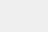# Kullback-Leibler散度(通常简称为KL散度)

KL散度### 我们分布的熵

KL散度起源于信息论。信息论的主要目标是量化数据中有多少信息。信息论中最重要的指标称为熵，通常表示为$H$。概率分布的熵的定义是：

$$H=-\sum_{i=1}^{N}p(x_i)logp(x_i)$$

### 使用KL散度测量丢失的信息

Kullback-Leibler散度只是对我们的熵公式的略微修改。不仅仅是有我们的概率分布p，还有上近似分布q。然后，我们查看每个log值的差异：

$$D_{KL}(p||q)=\sum_{i=1}^{N}p(x_i)(logp(x_i)-logq(x_i))$$

$$D_{KL}(p||q)=E[logp(x_i)-logq(x_i)]$$

$$D_{KL}(p||q)=\sum_{i=1}^{N}p(x_i)(log\frac{p(x_i)}{q(x_i)})$$

​​因为$loga-logb=log\frac{a}{b}$

### 比较我们的近似分布

$$D_{kl}(Observed ∣∣ Uniform)=0.338$$

$$D_{kl}(Observed ∣∣ Binomial)=0.477$$

### KL散度不是距离

$$D_{kl}(Binomial∣∣Observed)=0.330$$

### 使用KL散度进行优化$$[6,11]=\frac{p}{5};[0,5]=\frac{1-p}{6}$$